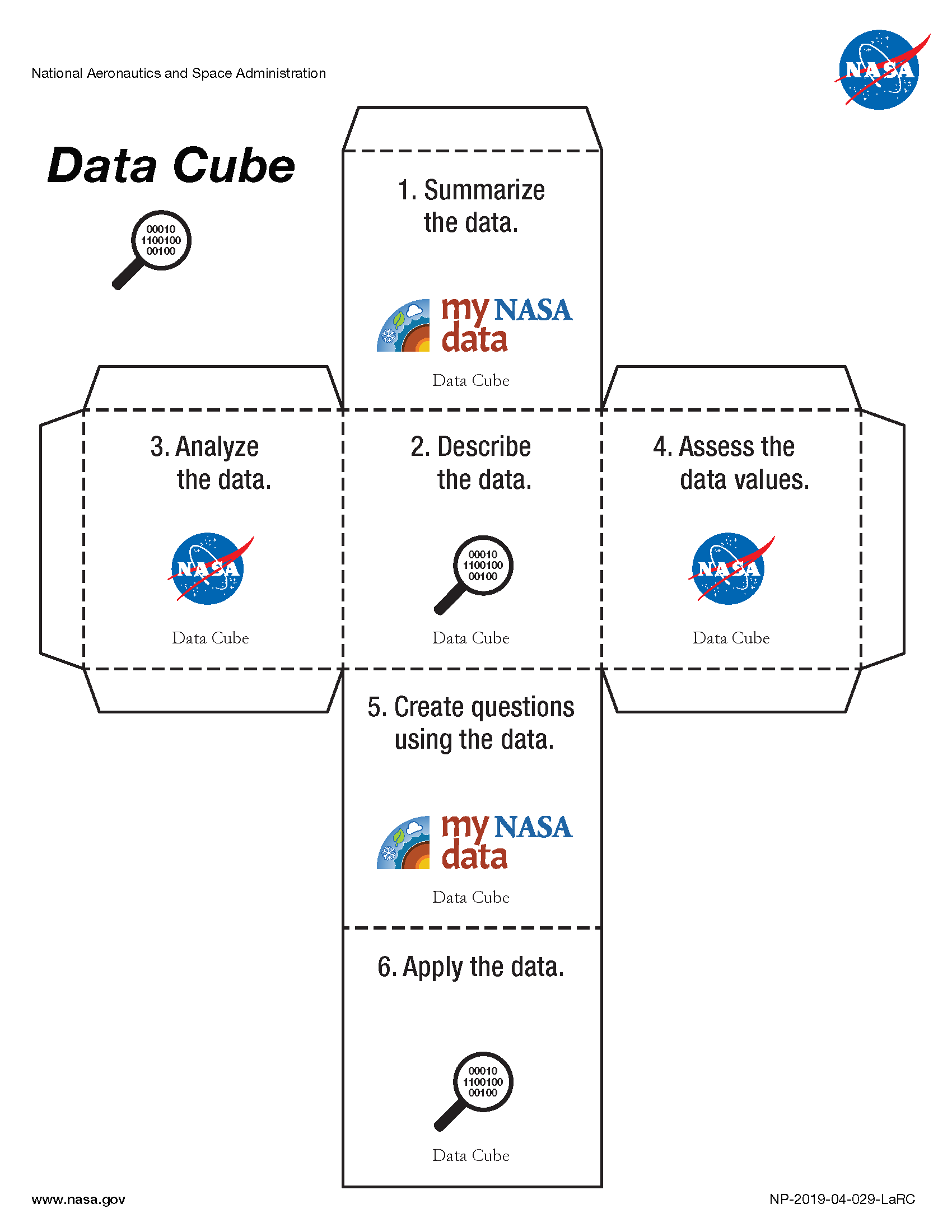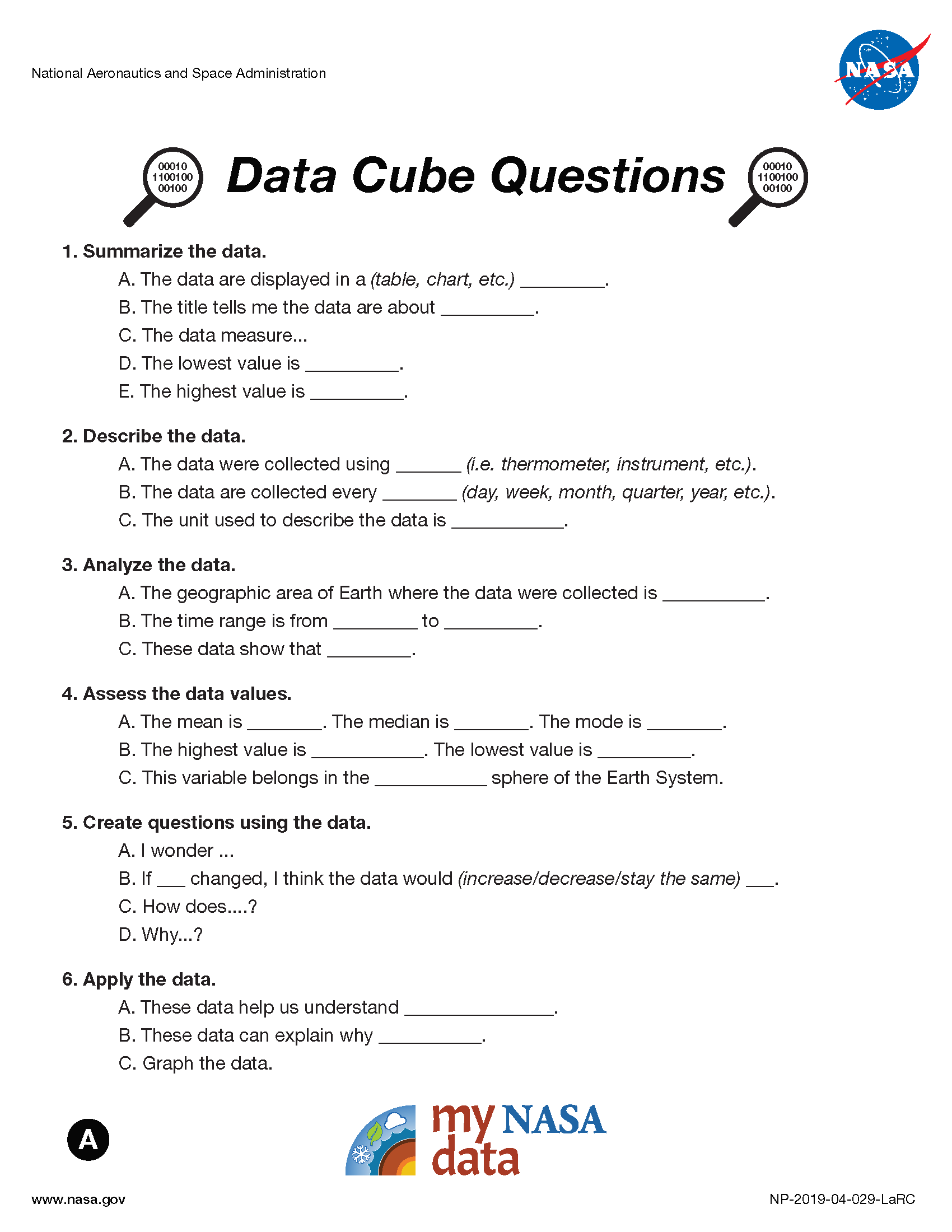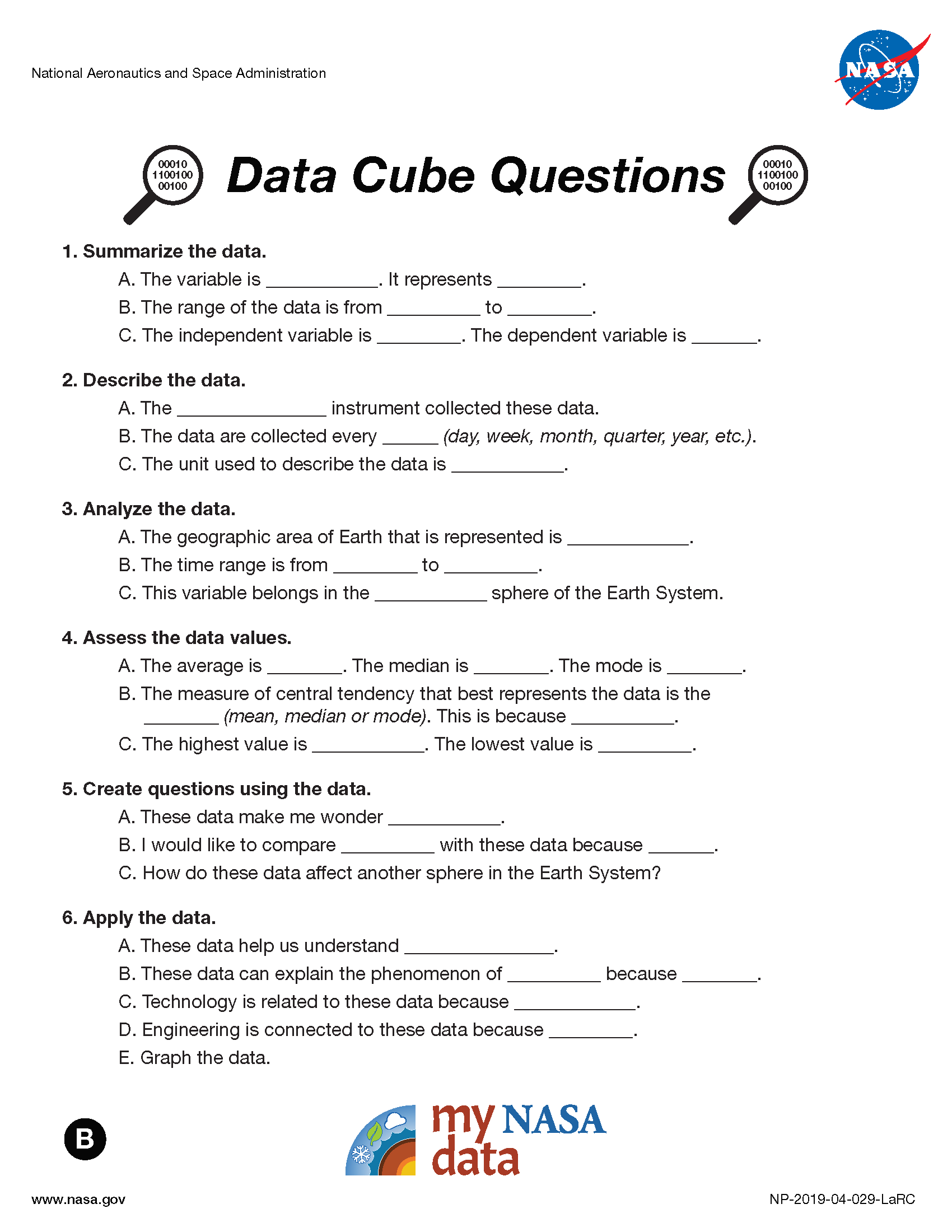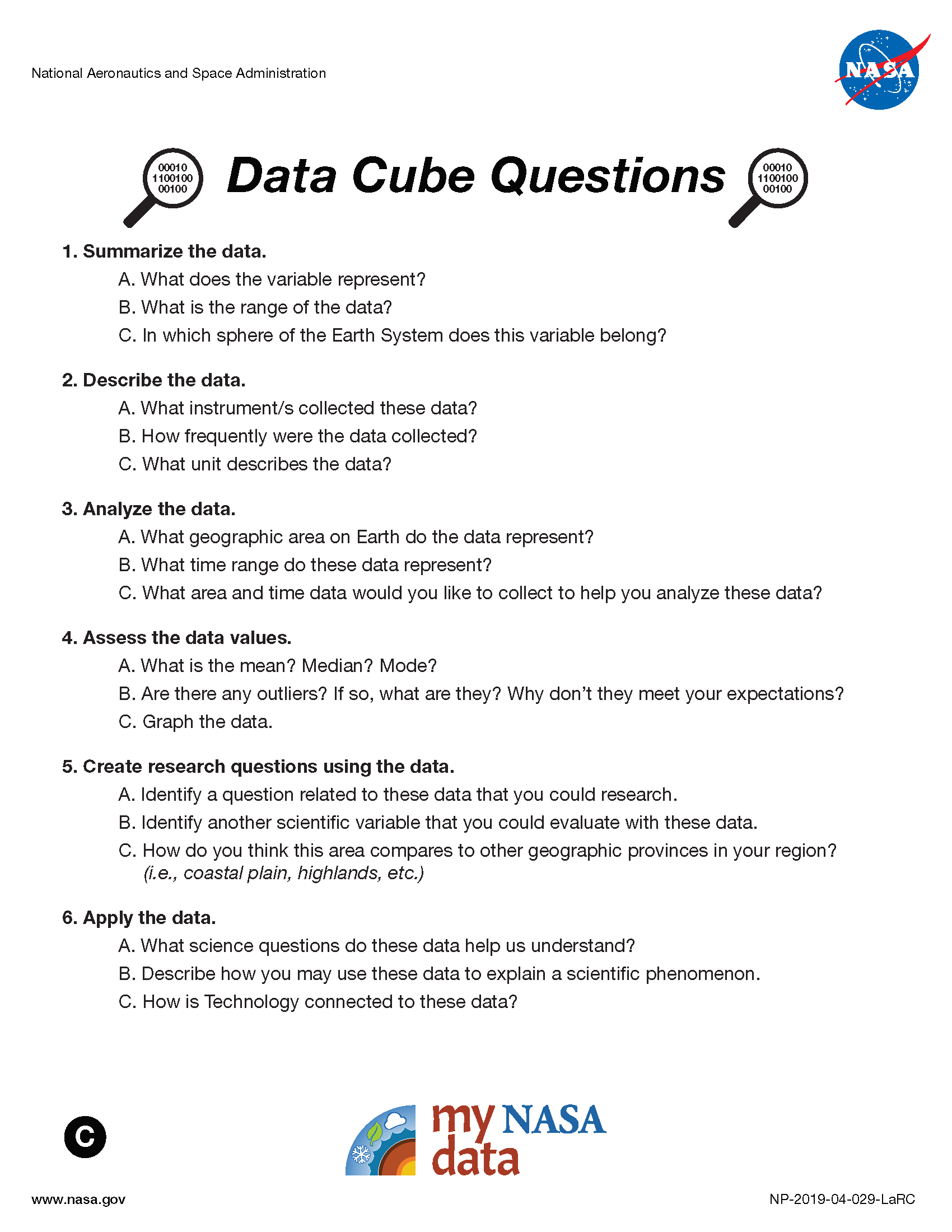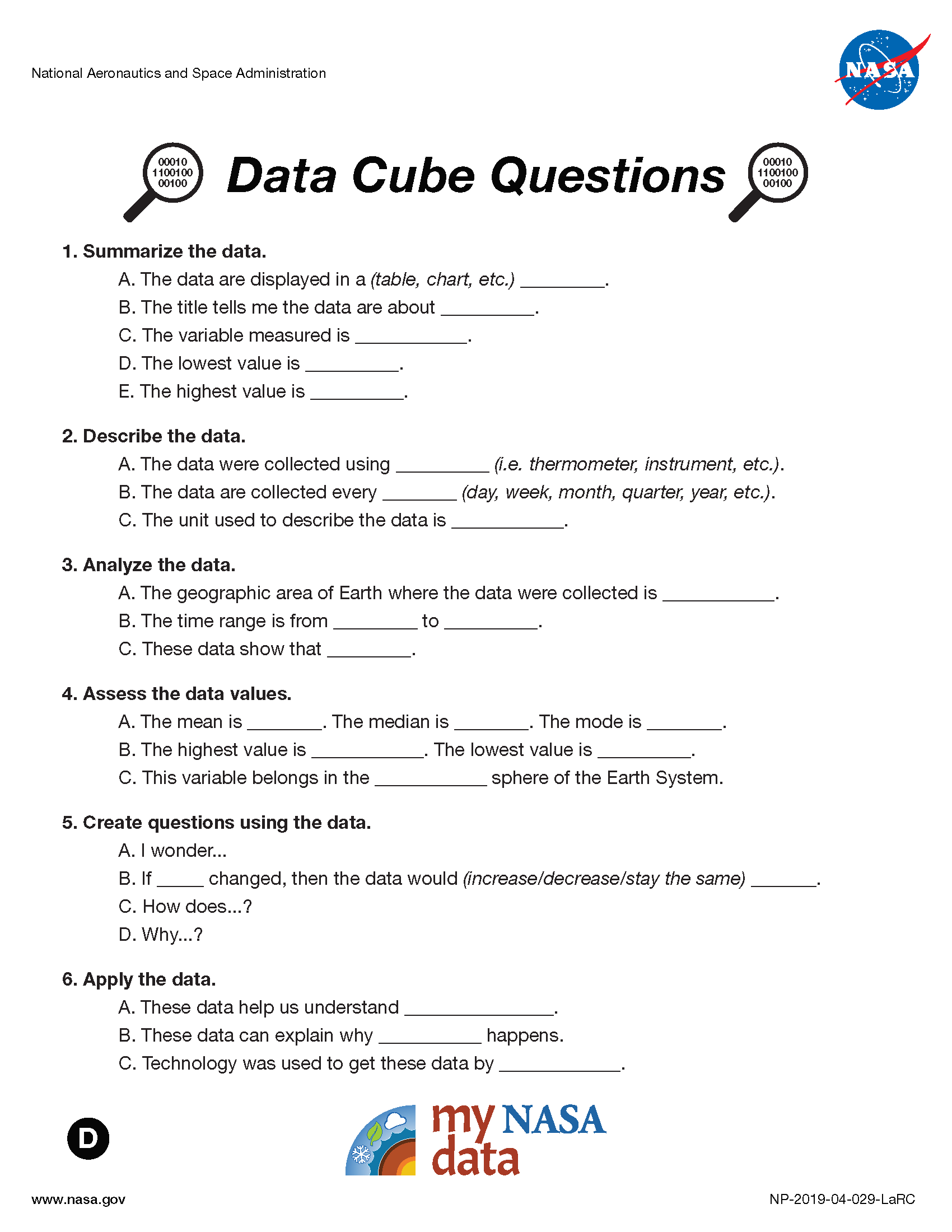# Using Mathematics and Computational Thinking with MND

Scientists and engineers use mathematics and quantitative thinking to representing variables, behaviors, and their relationships. Mathematics is used to create models and simulations; statistically analyze data; and recognize, communicate, and look for relationships with other variables.

Although there are differences in how mathematics and computational thinking are applied in science and in engineering, mathematics often brings these two fields together by enabling engineers to apply the mathematical form of scientific theories and by enabling scientists to use powerful information technologies designed by engineers. Both kinds of professionals can thereby accomplish investigations and analyses and build complex models, which might otherwise be out of the question. (NRC Framework, 2012, p 65)

K-2: Mathematical and computational thinking in K-2 builds on prior experiences and progresses to recognizing that mathematics can be used to describe the natural and designed world(s).

• Use counting and numbers to identify and describe patterns in the natural and designed world(s).
• Describe, measure, and/or compare the quantitative attributes of different objects and display the data using simple graphs.

3-5: Mathematical and computational thinking in 3-5 builds on K-2 experiences and progresses to extending quantitative measurements to a variety of physical properties and using computation and mathematics to analyze data and compare alternative design solutions.

• Organize simple data sets to reveal patterns that suggest relationships.

6-8: Mathematical and computational thinking in 6-8 builds on K-5 experiences and progresses to identifying patterns in large datasets and using mathematical concepts to support explanations and arguments.

9-12: Mathematical and computational thinking in 9-12 builds on K-8 experiences and progresses to using algebraic thinking and analysis, a range of linear and nonlinear functions including trigonometric functions, exponentials and logarithms, and computational tools for statistical analysis to analyze, represent, and model data. Simple computational simulations are created and used based on mathematical models of basic assumptions.

• Use digital tools (e.g., computers) to analyze very large datasets for patterns and trends.
• Use mathematical or computational representations of phenomena to describe explanations. (HS-ESS1- 4)(HS-ESS3- 6)
• Create a computational model or simulation of phenomena, designed device, process, or system. (HS-ESS3- 3)

Data Literacy Cubes

The Data Literacy Cubes can be used to foster mathematics and computational thinking. Cubes are available for analyzing maps, graphs and data. Each type of cube has differentiated questions to scaffold learners in their analysis.